# Practice questions with answers on heat and thermodynamics for JEE and Competitive examinations

In this page we have Practice questions with answers on heat and thermodynamics for JEE and Competitive examinations . Hope you like them and do not forget to like , social shar and comment at the end of the page.

Question 1: What increase in radiated power results when the temperature of a blackbody is increased from 7 to 287 0 C
a)4
b) 8
c) 16
d) None of these

Question 2: The internal energy of an ideal gas depends on
a) Temperature
b) Pressure
c) Volume
d) None of these

Question 3: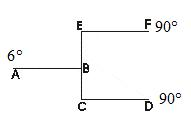All Rod are of equal length and equal area of crossection.Find the temperature at point B
a,     45
b,     51.4
c,     50
d,     51

Question 4:
An ideal gas is taken through a cyclic thermodynamics process through four steps.
The amount of heat involved in the steps are Q1 = 5960 J,Q2 = - 5600 J,Q3= -3000 J,Q4 = -3600 J
respectively. The corresponding quantities of Internal energy changes are ΔU1 = 3.760 J ,ΔU2 = - 4800 J,ΔU3 = -1800 J,ΔU4 = ?
find the value ΔU4 & net work done
a, 2930 J, 960 J
b, 2830 J, 900 J
C, 2930 J, -960 J
d, -2930 J, 960 J

Question 5:
4 moles of an ideal gas undergoes an isothermal expansion at temperature T during which the volume becomes η times.
W -> Workdone by the gas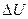-> Change in internal energy

a)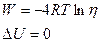b)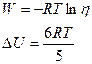c)d)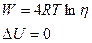Question 6 M moles  of a ideal polyatomic gas(Cv=7R/2)  are in cylinder at temperature T.A heat Q is suuplied to the gas. Some M/3 moles of the gas dissociated into atoms while temperature remains constant. Find the correct relation
a) 3Q=4MRT
b) 2Q=3MRT
c) Q=4MRT
d) 7Q=4MRT

Question 7 Absorbing power of the surface is .7. Transmitting power of the surface is .1
A total heat Q is incident on the surface. Find the heat reflected back?
a)Q/6
b)Q/4
c) Q/2
d) Q/5

Question 8
A hole of radius R1 is made centrally in a circular disc of thickness d and radius R2.The inner surface is maintained at temperature T1 and other surface is maintained at T2 (T1 > T2).Thermal conductivity of the circular plate is K.
a. Find the temperature as a function of radius from centre
b. Find the heat flow per unit time

Assertion and Reason
a) Statement I is true ,statement II is true ,statement II is correct explanation for statement I
b) Statement I is true ,statement II is true ,statement II is not a correct explanation for statement I
c) Statement I is true, Statement II is false
d) Statement I is False, Statement II is True

Question 9
STATEMENT 1:The molar specific heat capacity of the ideal gas in isothermal process is infinity
STATEMENT 2:Heat transfer is non zero in the Isothermal process

Question 10
STATEMENT 1:Equation of state for a real gas is (P+a/V2)(V-b)=nRT
STATEMENT 2: Molecular attraction is not neglible and the size of molecules are not neglibile in comparison to average separation between them